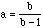Date: Sun, 4 Oct 1998 21:58:04 -0600 (CST) Subject: Math 8 - enriched Name: Emily Who is asking: Student Level: Middle Question: Find a pair of fractions that when they are multiplyed and added the sum /product is the same Hi Emily When I read your problem I thought of 1/2 and 1/3 since the product has 6 in the denominator and the common denominator is also 6. This doesn't work since 1/2 + 1/3 = 5/6 and 1/2 x 1/3 = 1/6 but 1/2 - 1/3 = 1/2 x (-1/3) = -1/6 so 1/2 and -1/3 satisfy your condition. If you consider 2/1 to be a fraction then 2/1 and 2/1 will also work, in fact if you know some algebra you can find many solutions. If a and b are any numbers for which ab = a + b then subtracting a from both sides gives ab - a = b. That is a(b - 1) = b orHence if you let b be any fraction (except b=1) andthen ab = a + b. For example if b = 1/4 then a = -1/3. Cheers, Penny

Go to Math Central